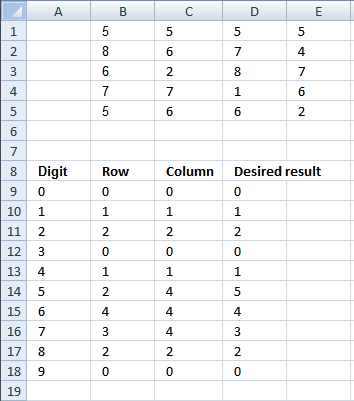Author: Oscar Cronquist Article last updated on January 11, 2019Question:
I have a question that I can’t seem to find an answer to:
I want to make a full count of digits 0 to 9 while ignoring duplicates in any line
B       C       D       E
5       5       5       5
8       6       7       4
6       2       8       7
7       7       1       6
5       6       6       2For example, with the digits shown above, my results for the count will be:
0=0; 1=1; 2=2; 3=0; 4=1; 5=5; 6=4; 7=3; 8=2; 9=0
The formula below does not work for the “5” count since it counts all the occurrences:
=COUNTIF(\$B\$1:\$E\$5,5)

I can't achieve the desired result for number 5. I calculated any line as "in any row" and "in any column". I guess the desired result for number 5 is a typo.

Array formula in cell B9:

=SUM(IF(FREQUENCY(IF(A9=\$B\$1:\$E\$5, ROW(\$B\$1:\$E\$5)-MIN(ROW(\$B\$1:\$E\$5))+1, ""), ROW(\$1:\$5))>0, 1, 0))

Copy cell B9 and paste down as far as needed.

Array formula in cell C9:

=SUM(IF(FREQUENCY(IF(A9=\$B\$1:\$E\$5, COLUMN(\$B\$1:\$E\$5)-MIN(COLUMN(\$B\$1:\$E\$5))+1, ""), ROW(\$1:\$4))>0, 1, 0))

Copy cell C9 and paste down as far as needed.

### How the formula works in cell B9

Step 1 - Find digit in array

=SUM(IF(FREQUENCY(IF(A9=\$B\$1:\$E\$5, ROW(\$B\$1:\$E\$5)-MIN(ROW(\$B\$1:\$E\$5))+1, ""), ROW(\$1:\$5))>0, 1, 0))

A9=\$B\$1:\$E\$5

becomes

0={5, 5, 5, 5;8, 6, 7, 4;6, 2, 8, 7;7, 7, 1, 6;5, 6, 6, 2}

becomes

{FALSE, FALSE, FALSE, FALSE;FALSE, FALSE, FALSE, FALSE;FALSE, FALSE, FALSE, FALSE;FALSE, FALSE, FALSE, FALSE;FALSE, FALSE, FALSE, FALSE}

Step 2 - Convert boolean array to row numbers

=SUM(IF(FREQUENCY(IF(A9=\$B\$1:\$E\$5, ROW(\$B\$1:\$E\$5)-MIN(ROW(\$B\$1:\$E\$5))+1, ""), ROW(\$1:\$5))>0, 1, 0))

IF(A9=\$B\$1:\$E\$5, ROW(\$B\$1:\$E\$5)-MIN(ROW(\$B\$1:\$E\$5))+1, "")

becomes

IF({FALSE, FALSE, FALSE, FALSE;FALSE, FALSE, FALSE, FALSE;FALSE, FALSE, FALSE, FALSE;FALSE, FALSE, FALSE, FALSE;FALSE, FALSE, FALSE, FALSE}, {1, 2, 3, 4}, "")

becomes

{"", "", "", "";"", "", "", "";"", "", "", "";"", "", "", "";"", "", "", ""}

Step 3 - Count row numbers

=SUM(IF(FREQUENCY(IF(A9=\$B\$1:\$E\$5, ROW(\$B\$1:\$E\$5)-MIN(ROW(\$B\$1:\$E\$5))+1, ""), ROW(\$1:\$5))>0, 1, 0))

FREQUENCY(data_array, bins_array)
Calculates how often values occur within a range of values and then returns a vertical array of numbers having one more element than Bins_array.

FREQUENCY(IF(A9=\$B\$1:\$E\$5, ROW(\$B\$1:\$E\$5)-MIN(ROW(\$B\$1:\$E\$5))+1, ""), ROW(\$1:\$5))

becomes

FREQUENCY({"", "", "", "";"", "", "", "";"", "", "", "";"", "", "", "";"", "", "", ""}, {1; 2; 3; 4; 5})

becomes

{0; 0; 0; 0; 0}

Step 4 - Count numbers larger than zero

=SUM(IF(FREQUENCY(IF(A9=\$B\$1:\$E\$5, ROW(\$B\$1:\$E\$5)-MIN(ROW(\$B\$1:\$E\$5))+1, ""), ROW(\$1:\$5))>0, 1, 0))

becomes

=SUM(IF({0; 0; 0; 0; 0}>0, 1, 0))

becomes

=SUM({0; 0; 0; 0; 0}) and returns 0 (zero).